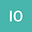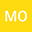Similarity of Hybrid Numbers
••• İskender Öztürk,
• MUSTAFA ÖZDEMİR
İskender Öztürk
Akdeniz University
Author ProfileMUSTAFA ÖZDEMİR
Akdeniz Universitesi
Author Profile## Abstract

The set of hybrid numbers is a noncommutative number system unified and generalized the complex, dual and double(hyperbolic) numbers with the relation $$\mathbf{ih}$$ $$=-\mathbf{hi}=\varepsilon+\mathbf{i.}$$ Two hybrid numbers $$\mathbf{p}$$ and $$\mathbf{q}$$ are said to be similar if there exist a hybrid number $$\mathbf{x}$$ satisfying the equality $$\mathbf{x}^{-1}\mathbf{qx}=\mathbf{p}$$. And it is denoted by $$p\sim q$$. In this paper, we study the concept of similarity for hybrid numbers by solving the linear equations $$\mathbf{px}=\mathbf{xq}$$ and $$\mathbf{qx}-\mathbf{xp=c}$$ for $$\mathbf{p},\mathbf{q},\mathbf{c}\in\mathbb{K}\mathbf{.}$$

Keywords : Hybrid numbers, quaternions, coquaternions, dual and double numbers.

MSC Classification : 15A66, 11R52, 15A18, 15A06, 16S50.

#### Peer review status:ACCEPTED

29 Jan 2020Submitted to Mathematical Methods in the Applied Sciences
03 Feb 2020Submission Checks Completed
03 Feb 2020Assigned to Editor
15 Feb 2020Reviewer(s) Assigned
09 May 2020Review(s) Completed, Editorial Evaluation Pending
12 May 2020Editorial Decision: Revise Minor